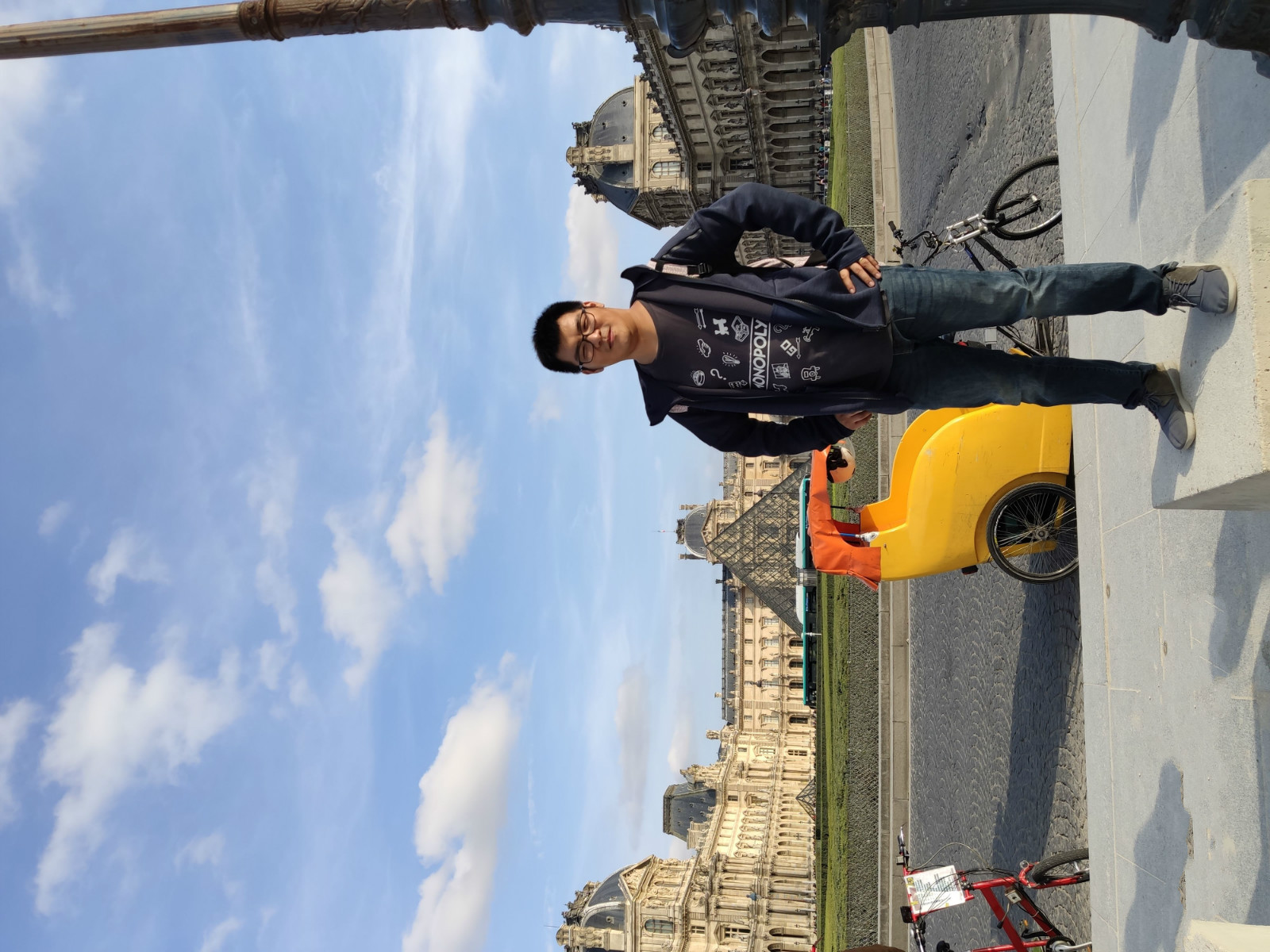## Harbin Engineering UniversityCollege of Mathematical SciencesAssociate Professor
College of Mathematical Sciences
Harbin Engineering University
Harbin, China
Office:
e-mail: qinchao@hrbeu.edu.cn

## Publications

### Papers

• On the solvability of regular subgroups in the holomorph of a finite solvable group (joint with with C. Tsang)
International Journal of Algebra and Computation, 30(02), 253--265, (2020).(pdf)

• $K1$-congruences for three-dimensional Lie groups (joint with Daniel Delbourgo)
Annales mathématiques du Québec, 43(1), 161--211, (2019). (pdf)

• On $\lambda$-invariants attached to cyclic cubic number fields (joint with Daniel Delbourgo)
LMS Journal of Computation and Mathematics, 18(1), 684--698, (2015). (pdf)

### Other Writing

• Iwasawa theory over solvable three-dimensional $p$-adic Lie extensions
PhD thesis, University of Waikato (pdf)

• Zeroes of $5$-adic and $7$-adic $L$-functions over cubic fields
Honours dissertation, University of Waikato (pdf)